# Pharmacokinetics

3
Pharmacokinetics

Jim E. Riviere

Pharmacokinetics is best defined as the use of mathematical models to quantitate the time course of drug absorption and disposition in man and animals. With the tremendous advances in medicine and analytical chemistry, coupled with the almost universal availability of computers, what was once an arcane science has now entered the mainstream of most fields of human and veterinary medicine. This discipline has allowed dosages of drugs to be tailored to individuals or groups to optimize therapeutic effectiveness, minimize toxicity, and avoid violative tissue residues in the case of food-producing animals. This subject and its concepts have become especially important as a consequence of the dramatic and almost radical changes that occurred at the end of the last decade relative to the regulations surrounding drug use in veterinary medicine in the United States. For most of the recent past, the operative concept was that a single dose of drug listed on a product label was optimal for all therapeutic uses. Recently, however, the legal concept of “flexible or professional labeling” and the passage by the US Congress in 1994 of the Animal Medicinal Drug Use Clarification Act (AMDUCA) legalizing extralabel drug use challenged this simplistic ideal of a single optimal dose. The veterinarian must now select a drug dose based on numerous factors inherent to the therapeutic scenario at hand to maximize therapeutic efficacy and minimize the likelihood of drug-induced toxicity or induction of microbial resistance. Furthermore, linking pharmacokinetic models to models of drug action, presented in the next chapter, allows for developing a quantitative link between dose and efficacy or toxicity. Unlike human medicine and companion animal practices, food animal veterinarians face the further restriction that proper withdrawal times must be determined to ensure that drug residues do not persist in the edible tissues or by-products (milk, eggs) of treated animals long after they have left the care of the veterinarian (Figure 3.1). As will be demonstrated and further discussed in Chapter 61, the “withdrawal time” is in reality a pure pharmacokinetic parameter since it can be calculated solely from a knowledge of the legal tissue tolerance and the drug’s half-life or rate of decay in that tissue.

Yet it is not only the food animal veterinarian that faces these challenges. The laboratory animal and exotic/zoo animal worker must often extrapolate drug dosages across species with widely differing body sizes and physiology since there are very few approved drugs for the treatment of such animals. Pharmacokinetic principles and techniques are ideally suited for this application. Practitioners are often faced with disease processes (e.g., renal failure) that are known to affect the disposition of a drug. Knowledge of how such a pathological process affects a drug’s clearance is sufficient knowledge to adapt a dosage regimen appropriate for this condition.

The previous chapter of this text presented the underlying physiology of drug fate. The processes involved in absorption, distribution, metabolism, and elimination are the primary phenomena that must be quantitated to predict the fate of a drug or toxicant in an animal. The two primary characteristics needed to adequately describe these processes are their rate and extent. In fact, this can be appreciated in the origin of the word kinetic, which is defined as: “of or resulting from motion.” Many mathematical approaches to this problem have evolved over the course of the history of pharmacokinetics. The reader is suggested to consult the selected readings for more in depth presentations of pharmacokinetic modeling. In addition, hybrid as well as novel strategies are constantly being developed to quantitate these processes. However, all approaches share certain fundamental properties that are based upon estimating the rates of chemical movement.

## A Primer on the Language of Pharmacokinetics

The roots of pharmacokinetics lie in the estimation of rates. The language is that of differential calculus. It is instructive to briefly overview the basic principles of rate determination since the logic imbedded in its syntax forms the basis of pharmacokinetic terminology.

To begin, a rate in pharmacokinetics is defined as how fast the mass of a compound changes per unit of time, which is expressed mathematically as the change (represented by the Greek letter delta, Δ) in mass per small unit of time (Δt). This is synonymous with the flux of drug in a system. Units of rate are thus mass/time. For the sake of convenience only, we will express this in terms of mg/min. We will begin this discussion using mass of a compound (X), which in clinical terms would be related to the dose, rather than using concentration. As will be developed shortly, mass and concentration are easily convertible using the proportionality factor of volume of distribution.

The rate of drug excretion ΔX/Δt actually has two components, a constant that reflects the rate of the process and the amount of compound available for transfer (Equation 3.1):

(3.1)where K is the fractional rate constant (1/min), X (mg) is the mass or amount of a compound available for transfer by the process being studied, and n is the order of the process.

For a first-order process, n = 1. Since X1 = X, this equation simplifies to Equation 3.2:

(3.2)By definition, in this first-order (n = 1) or linear processes, K is constant and thus the actual rate of the process (ΔX/Δt) varies in direct proportion (and hence linearly) to X. K can be viewed as the fraction of X that moves in the system being studied (absorbed, distributed, or eliminated) per unit of time. Therefore, as X increases, ΔX/Δt increases in direct proportion. In linear models, the rate constant is fixed but the rate of the process changes in direct proportion to the mass available for movement.

As can again be appreciated by examining the equation for Fickian diffusion (Equation 2.1 in Chapter 2), compounds that are either absorbed, distributed, or eliminated in direct proportion to a concentration gradient are by definition first-order rate processes. The rate constants (Kn) modeled in pharmacokinetics are actually aggregate constants reflecting all of the membrane diffusion and transfer processes involved in the disposition parameter being studied. This includes pH partitioning phenomena in the body, which exist when blood and a cellular or tissue compartment have a pH gradient that alters the fraction of drug available for diffusion. Recall that it is only the unionized fraction of a weak acid or base that diffuses down its gradient across a lipid membrane. The rate constant also reflects the degree of plasma protein binding since only the free fraction of drug is available for distribution. The actual value of a K in a pharmacokinetic model thus reflects all of these variables whose relationship defines the biological system that we are attempting to quantitate.

For a nonlinear or zero-order process, by definition n = 0. Since X0 = 1, the rate equation now becomes

(3.3)In this scenario, the rate of excretion is fixed and thus independent of the amount of compound available, X. Ko now has the units of rate (mg/min) and is not a mass-independent fractional rate constant. Although this would appear to simplify the situation, in reality nonlinear kinetics actually complicate most models. Nonlinear behavior becomes evident when saturation of a process occurs. The focus of most pharmacokinetic studies is on drugs with linear pharmacokinetics since the majority of therapeutically active compounds are described by these models.

The use of ΔX/Δt to describe the rate of a process is experimentally and mathematically cumbersome, since ΔX/Δt changes as a function of concentration. Figure 3.2 graphically depicts this scenario. Calculus has been used to describe these same processes using the concept of a derivative. Instead of describing rates in terms of some small, finite time interval (Δt), differential equations express rate in terms of the change in compound mass over an infinitesimally small time interval termed dt. Equation 3.1 could now be written as the following (Equation 3.4):

(3.4)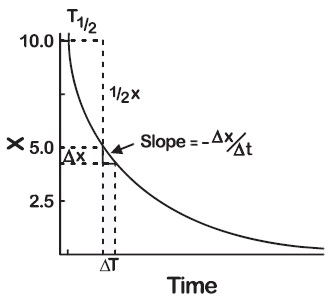Figure 3.2 Plot of the decay in drug (X) versus time. The T1/2 is defined as the time required for X to deplete to 1/2 X. Slope at any time is ΔX/ΔT.

The biological interpretation is identical. Note that K and X are the same in both equations; the only change is a conceptual one in that dX/dt now describes the instantaneous rate of change in mass over time. By convention, if the amount of drug is increasing, dX/dt is positive (e.g., absorption of drug into the blood); if it is declining (e.g., elimination or distribution from the blood), the rate is negative or −dX/dt.

One may solve a differential equation through the process of integration (∫), which transforms the equation back into terms of t rather than dt. Integration is in reality a process by which the area under the curve (AUC) defined by ΔX/Δt is taken. By repeatedly summing these areas for the entire experimental period, the area under the curve will be obtained. We introduced this concept in Equation 2.4 when the concept of AUC was introduced. Analogous to the relation of a derivative to a slope, integration sums the areas under infinitesimally small regions defined by dX/dt.

We can use the technique of integration to solve the rate Equation 3.4. We must integrate the equation from X at time zero (X0) through X at time t (Xt) to obtain a formula for the mass of drug at any time (Equation 3.5):

(3.5)There are numerous techniques to accomplish this integration and the interested reader should consult a calculus textbook for further details. The result is the following (Equation 3.6):

(3.6)where e is the base of the natural logarithm (e = 2.713). It is important to realize that the process of integrating the differential equation describing rate generates the exponential term found in most linear pharmacokinetic models. Exponentials can easily be eliminated from an equation by taking their natural logarithm (ln) since the logarithm is defined as the power to which a base (in this case e) is raised. Taking the natural logarithms of Equation 3.6 yields the following (Equation 3.7):

(3.7)If one plots these data, a straight line results, as seen in Figure 3.3, which is much easier to deal with than the curve in Figure 3.2. Recalling the algebraic expression for a straight line on x–y coordinates, in this case the y intercept becomes X0 and the slope of the line is −K. The equation has been linearized providing a simple graphical method to calculate the rate constant.

This equation can be linearized because it is a first-order rate function. This type of plot, which is widely used throughout pharmacokinetics, is termed a semilogarithmic plot (in contrast to the Cartesian plot) since the logarithm of mass is plotted against time. Again, when a straight line results on a semilogarithmic plot, one can assume that a linear first-order process is operative and the slope of the line is the exponent of an exponential equation. Alternatively, a linear regression program on a computer or calculator could be used to calculate K by regressing the ln X against time; the slope is −K and the y intercept is lnX0. In earlier work, graph paper was used that plotted logarithms to the base 10 (log x) {10x} rather than the base e (ln x) {ex} where x is the logarithm, the transformation of bases can be accomplished as the following (Equation 3.8):

(3.8)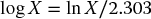which transforms Equation 3.7 to

(3.9)If base 10 semilogarithmic graph paper is used to plot Figure 3.3, the slope becomes −K/2.303. As this technique was in widespread use before the advent of digital computers, this is still encountered in older manuscripts and texts.

## The Concept of Half-Life

The exponential equations in pharmacokinetics have another property that is important to biological applications. This is the concept of half-life (T1/2) whose logic is central to much of this discipline. The astute biologist reading this text will have realized that Equation 3.6 is the same as that used to describe population doubling times in microbiology or ecology and used to generate population growth curves, defined as the time needed for a population of organisms to double their total numbers when they are in their so-called logarithmic growth phase. The only difference is that since growth is described, the exponent is positive in this application. In pharmacokinetics, our perspective is decay with a T1/2 instead being the time required for the amount of drug to decrease by one-half or 50%. The concept of T1/2 is applicable only to first-order rate processes.

Using Equation 3.7, one can derive a simple equation for T1/2. We first rearrange terms to solve for T, which yields

(3.10)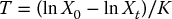We now solve for the time at which Xt is equal to 1/2 the initial amount X0, that is, where T = T1/2. Substituting these values, the equation reduces to

(3.11)It is this transformation of K with T1/2 that introduces the ln 2 or 0.693 into many pharmacokinetic equations.

What does T1/2 really mean? Assume that we start with X, decrease it by half, and repeat this process 10 times. Table 3.1  compiles this data and lists how much drug is remaining and how much has been excreted over each Δt corresponding to one T1/2. Note that if you sum these columns, you would have accounted for 99.9% of the original dose X. After 10 T1/2s, 99.9% of the drug has been eliminated or the rate process being studied has been completed. This also illustrates the logic that must be used when dealing with doses. For example, if you double the dose to 2×, then after one T1/2 you would be back to the original dose! Many rules of thumb used in pharmacokinetics and medicine are based on this simple fact. For therapeutic drugs, most workers assume that after five T1/2s, the drug has been depleted or the process is over since 97% of the depletion has occurred. This also illustrates a very simple way to calculate T1/2, by simply determining the time required for drug concentration to decrease by 50%. This was depicted in Figure 3.2. Equation 3.11 can then be used to obtain K. It now is time to develop our first pharmacokinetic model using mathematical rather than physiological concepts.

## One-Compartment Open Model

The most widely used modeling paradigm in comparative and veterinary medicine is the compartmental approach. In this analysis, the body is viewed as being composed of a number of so-called equilibrium compartments, each defined as representing nonspecific body regions where the rates of compound disappearance are of a similar order of magnitude. Specifically, the fraction or percent of drug eliminated per unit of time from such a defined compartment is constant. Such compartments are classified and grouped on the basis of similar rates of drug movement within a kinetically homogeneous but anatomically and physiologically heterogeneous group of tissues. These compartments are theoretical entities that allow formulation of mathematical models to describe a drug’s behavior over time with respect to movement within and between compartments. Since pharmacologists and clinicians sample blood as a common and accessible biological matrix for assessing drug fate, most pharmacokinetic models are constructed with blood or plasma drug concentrations as the central reference to which other processes are related.

The simplest compartment model is when one considers the body as consisting of a single homogeneous compartment; that is, the entire dose X of drug is assumed to move out of the body at a single rate. This model, depicted in Figure 3.4, is best conceptualized as instantly dissolving and homogeneously mixing the drug in a beaker from which it is eliminated by a single rate process described by the rate constant K, now termed Kel. Since the drug leaves the system, the model is termed open. Equation 3.6 is the pharmacokinetic equation for the one-compartment open model. Although expressed in terms of the amount of drug remaining in the compartment, most experiments measure concentrations. This requires the development of the volume of distribution (Vd) (recall Equation 2.5 when distribution was discussed). In terms of the one-compartment model, this would be the volume of the compartment into which the dose of drug (D) instantaneously distributes. Vd thus becomes a proportionality factor relating D to the observed concentration Cp by

(3.12)Using this relation, we can now rewrite Equation 3.6 in terms of concentrations, which are experimentally accessible by sampling blood, instead of the total amount of drug remaining in the body.

(3.13)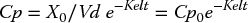A semilogarithmic plot seen after intravenous administration using this model is depicted in Figure 3.5. Vd quantitates the apparent volume into which a drug is dissolved, since, recalling the discussion in Chapter 2, the true volume is determined by the physiology of the animal, the relative transmembrane diffusion coefficients, and the chemical properties of the drug being studied. A drug that is restricted to the vascular system will have a very small Vd; one which distributes to total body water will have a very large Vd. In fact, it is this technique that is used to calculate the plasma and interstitial spaces.

From this simple analysis, and using the model in Figure 3.4, a number of useful pharmacokinetic parameters may be defined. Assuming that an experiment such as depicted in Figure 3.5 has been conducted using a dose of D and values for Kel and Vd have been determined, T1/2 can easily be calculated from Equation 3.11 above.

### Clearance

Recalling the development of clearance concepts in Chapter 2, we now can easily determine ClB using this information. Clearance was defined as the volume of blood cleared of a substance by the kidney per unit of time. If one considers the whole body, this would read as the volume of distribution of drug in the body cleared of a substance per unit of time. Translating this sentence to the syntax of pharmacokinetic terminology and considering whole body elimination, Vd represents the volume and Kel the fractional rate constant (units of 1/time). Thus clearance is

(3.14)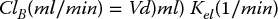There is another method available to calculate ClB. In Chapter 2, clearance was also defined in Equation 2.8 as the rate of drug excretion relative to its plasma concentration. We can also express this sentence in the syntax of pharmacokinetics and get this relation:

(3.15)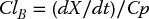If we integrate both the numerator and denominator of this relation from time 0 → ∞, the numerator is the sum of the total amount of drug which has been excreted from the body; that is, assuming intravenous administration, the administered dose D. The denominator is the integral of the plasma concentration time profile the area under the curve (AUC). The relation thus becomes:

(3.16)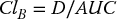There are two approaches to calculate AUC. A common approach is to use the trapezoidal method depicted in Figure 3.6. However, for the one-compartment model that generates the semilogarithmic C-T plot depicted in Figure 3.5, the problem is simply determining the area of the right triangle. The area of this triangle (AUC) is height divided by the slope of the hypotenuse, or:

(3.17)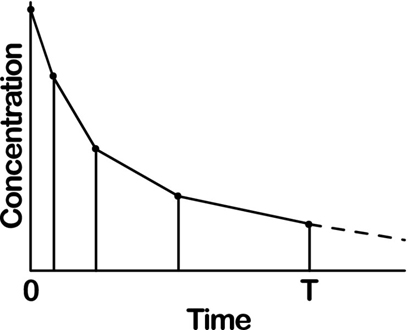Figure 3.6 Trapezoids formed from sampled concentration versus time data for calculation of areas. To estimate the total AUC, the curve must be extrapolated beyond the ultimate sample time, T, to infinity (—).

### Interpretation of Pharmacokinetic Parameters

With these equations, we now have the three so-called primary pharmacokinetic parameters describing drug disposition in the body: T1/2, ClB, and Vd. The data required to calculate them is a knowledge of dose and an experimental derivation of either Kel or T1/2.

This is a good place to discuss the limits of calculating parameters from such simple concentration-time profiles. Only two parameters are actually being “measured” from this analysis: the slope Kel and intercept Cp0 of the semilogarithmic plot, which – using Equation 3.12 directly – determines Vd. The third parameter Cl is “calculated” from the two measured parameters. Based on the mathematical method used to calculate these, some workers suggested that Kel and Vd are the independent parameters in a pharmacokinetic analysis and Cl is a derived parameter. This assertion is usually made when the statistical properties of the parameters are being defined since errors for these can be easily obtained. However, this belief is an artifact of the use of a compartmental model as a tool to get at values for these physiological parameters. Biologically, the truly independent parameters are the Vd and Cl, with Kel and thus T1/2 becoming the dependent variables. From this biological perspective, the true relationship is

(3.18)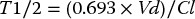The observed half-life of a drug is dependent upon both the extent of a drug’s distribution in the body and its rate of clearance. If the clearance of a drug is high (e.g., rapidly eliminated by the kidney), the T1/2 is relatively short. Logically, a slowly eliminated drug will have a prolonged T1/2. Not obvious at first is that if a drug is extensively distributed in the body (e.g., lipid-soluble drug distributed to fat), Vd will be large and the T1/2 will also be relatively prolonged. In contrast, if a drug has restricted distribution in the body (e.g., only the vascular system), the Vd will be small, a large fraction of the drug will be available for elimination, and thus the T1/2 relatively short. In a disease state, T1/2 may be prolonged by either a diseased kidney, a reduced capacity for hepatic drug metabolism, or an inflammatory state, which increases capillary perfusion and permeability, thus allowing drug access to normally excluded tissue sites. Therefore, T1/2 is physiologically dependent on both the volume of distribution and clearance of the drug.

ClB is the sum of clearances from all routes of administration:

(3.19)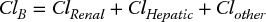There is another strategy that can be used to estimate clearance in an intravenous study. This is based on the basic principle of mass balance. The strategy is to infuse a drug into the body at a constant rate Ro (mass/time) and then measure plasma drug concentrations. By definition, when a steady-state plasma concentration is achieved, Css (mass/volume), the rate of drug input must equal the rate of clearance from the body, ClB:

(3.20)Rearranging this equation gives a simple formula for determining Cl:

(3.21)The Cl calculated in this manner is identical to that determined using Equations 3.14 and 3.16 above, and requires only knowing the rate of infusion and assaying the achieved steady-state concentration. One may also calculate the Vd from an intravenous infusion study by the relation:

(3.22)Many of the pharmacokinetic parameters above may also be obtained by analysis of urine data alone, an approach beyond the focus of the present introduction.

### Absorption in a One-Compartment Open Model

The analysis above assumes that the drug was injected into the body, which behaves as a single space into which the drug is uniformly dissolved. The first real-world complication is when the drug is administered by one of the extravascular routes discussed in Chapter 2. In this case, the drug must be absorbed from the dosing site into the bloodstream. The resulting semilogarithmic concentration–time profile, depicted in Figure 3.7, now is characterized by an initial rising component that peaks and then undergoes the same log-linear decline. The proper pharmacokinetic model for this scenario is depicted in Figure 3.8. The rate of the drug’s absorption is governed by the rate constant Ka. When the absorption process is finally complete, elimination is still described by Kel as depicted in Figure 3.5. The overall elimination half-life can still be calculated using Kel if this terminal slope is taken after the peak (Cmax) in the linear portion of the semilogarithmic plot (providing Ka ≫ Kel). However, calculation of Vd and Cl becomes more complicated since Ka is present and, unlike an intravenous injection, one is not assured that all of the drug has been absorbed into the body. In order to handle this, we must now write the differential equations to describe this process by including rate constants for absorption and elimination:

(3.23)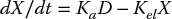where D is the administered dose driving the absorption process and X is now the amount of dose absorbed and available for excretion. The relationship between D and X is the absolute systemic availability F originally introduced in Equation 2.4 [X = FD]. In the language of differential equations, rates are simply additive, which allows the same data sets to be described in components reflecting the different processes. As above, integrating this equation and expressing it in terms of concentrations, gives the expression that describes the profile in Figure 3.9:

(3.24)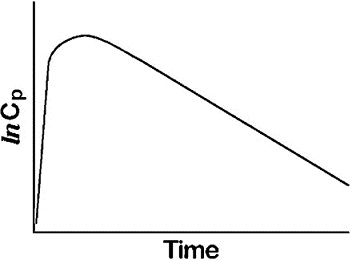Figure 3.7 Semilogarithmic plot of plasma concentration versus time with first-order absorption.Figure 3.8 One-compartment open pharmacokinetic model with first-order absorption.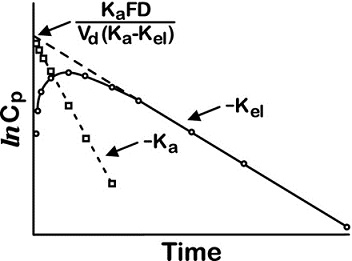Figure 3.9 Semilogarithmic plot of plasma concentration versus time using a one-compartment open pharmacokinetic model with first-order absorption. The profile is decomposed into two lines with slopes −Ka and −Kel.

This is an excellent point in the discussion to appreciate the validity of the use of multiexponential equations to describe blood C-T profiles as the exponential terms, which, like the rates above from which they were derived, are simply additive. A C-T profile is the sum of the underlying exponential terms describing the rate processes involved. This property of superposition is the basis upon which observed C-T profiles may be “dissected” to obtain the component rates. Figure 3.9 illustrates this process where an observed semilogarithmic profile is plotted as a composite of its absorption phase (controlled by Ka) and the elimination phase (controlled by Kel). In contrast to the intravenous scenario, the time zero intercept is now a more complex function, which is dependent upon the fraction of administered dose that is systemically available and thus able to be acted on by the elimination process described by the rate constant Kel. For this procedure to work, Ka must be greater than Kel so that at later time points e−Kat approaches zero. If Ka < Kel, the same C-T profile will result; however, now the terminal slope will be Ka as it is the rate-limiting process! One just “flip-flopped” Ka for Kel. In fact, recalling the discussion in Chapter 2 on slow-release dosing formulations, we termed the resulting effect on disposition of drug in the body an example of the flip-flop phenomenon, the origin of which is this relation. When an extravascular route of administration is used, one can never be certain that the C-T profile is not dependent upon a slow, and thus rate-limiting, absorptive process secondary to a formulation factor. If a depot or slow-release formulation is administered such that Ka < Kel, the terminal slope will reflect the rate of absorption rather than the rate of elimination. T1/2 may be overestimated as it will now reflect 0.693/Ka rather than 0.693/Kel. Complete absorption also cannot be assured (e.g., F = 1); thus one never truly knows the size of the absorbed dose. Accurate estimates of Cl and Vd, reflecting the true pharmacokinetic disposition of a drug, are required as input to determine these relations. These are best calculated after a complete intravenous injection. Finally, as discussed above for intravenous administration, urine analysis may also be used to estimate absorption parameters.

As we leave this section, it is a good time to underscore the relationship of some ADME processes as they translate to pharmacokinetic parameters; namely absorption, clearance, and volume of distribution, and further relate to the main determinants of a dosage regimen – namely, F and T1/2. This can be appreciated as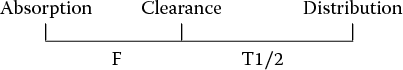F was determined by Equation 2.4 as the ratios of AUCoral/AUCiv. The intravenous D is a function of Cl and AUC from Equation 3.16. Half-life was a function of Vd and Cl in Equation 3.18. This scheme shows how F is a function of absorption and clearance processes (AME) while half-life is a function of clearance and distribution (DME).

Why are these relationships important? One of the main clinical applications of pharmacokinetic principles is to construct dosage regimens, the approach that is fully presented below. Diseases that change any of these primary pharmacokinetic parameters would be expected to change the plasma concentrations achieved after dosing, and thus drug effect. For example, renal disease that reduced GFR, might reduce ClB for drugs primarily eliminated by the kidney. Similarly, liver disease might alter disposition of drugs cleared by the liver. In contrast, diseases that resulted in severe elimination and fluid accumulation could alter Vd. Both of these scenarios would increase T1/2 and alter plasma concentrations. The relationship between concentration and effect is extensively discussed in the next chapter on pharmacodynamics.

## Two-Compartment Models

Many drugs are not described by a simple one-compartment model since the plasma concentration time profile is not a straight line. This reflects the biological reality that for many drugs, the body is not a single homogeneous compartment, but instead is composed of regions that are defined by having different rates of drug distribution. Such a situation is reflected in the two-compartment model depicted in Figure 3.10. The drug initially is distributed in the central compartment and by definition is eliminated from this compartment. The difference comes because now the drug also distributes into other body regions at a rate that is different from that of the central compartment.Figure 3.10 Generalized open two-compartment pharmacokinetic model after intravenous administration with elimination (Kel) from the central compartment. K12 and K21 represent intercompartmental micro-rate constants.

As presented in Chapter 2, there are many factors that determine the rate and extent of drug distribution into a tissue (e.g., blood flow, tissue mass, blood/tissue partition coefficient, etc.). When the composite rates of these flow and diffusion processes are significantly different than Kel, then the C-T profile will reflect this by assuming a biexponential nature. For many drugs, the central compartment may consist of blood plasma and the extracellular fluid of highly perfused organs such as the heart, lung, kidneys, and liver. Distribution to the remainder of the body occurs more slowly, which provides the physiological basis for a two-compartment model. Such a peripheral compartment is defined by a distribution rate constant (K12) out of the central compartment and a redistribution rate constant (K21) from the peripheral back into the central compartment. As discussed in the distribution chapter, depots or sinks may also occur. This is a pharmacokinetic concept where the distribution rate constants are significantly slower than Kel and thus become the rate-limiting factor defining the terminal slope of a biexponential C-T profile, a situation analogous to flip-flop in absorption studies.

We will begin the discussion of multicompartmental models with the principles of analyzing a two-compartment model after intravenous administration (Figure 3.11). This is the most common scenario encountered in comparative medicine and the principles easily translate to more complicated models. The fundamental principle involved is that the observed serum concentration time profile is actually the result of two separate pharmacokinetic processes that can be described by two separate exponential terms, commonly written as:

(3.25)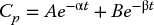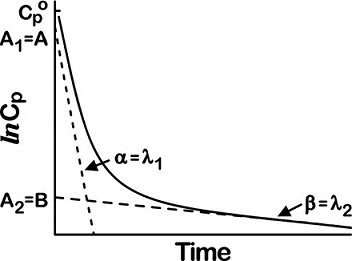Figure 3.11 Semilogarithmic plasma concentration versus time profile of a drug described by a two-compartment open model. Parameters are defined in the text.

Note the similarity of this biexponential equation to that presented for absorption in Equation 3.24. In this case we have terms with slopes (α and β) and corresponding intercepts (A and B). The C-T profile on semilogarithmic plots is depicted in Figure 3.11. By definition, α ≫ β and thus β is the terminal slope. If α ≈ β, the slopes of the two lines would be equal and we would be back to the single line of Figure 3.5 and a one-compartment model!

When dealing with multicompartmental models, it becomes necessary to introduce new nomenclature to denote the intercept terms and slopes of the C-T profile because, as will be shown shortly, the observed slopes are no longer synonymous with the elimination and distribution micro-rate constants as they were when we were analyzing absorption plots for Kel and Ka. When these models were constructed, the defining differential rate equations could be written in terms of the mass of drug in the central compartment (X). In the two-compartment model of Figure 3.10, this equation now must describe drug movement in terms of the mass of drug in compartment one and two. The solution to these differential equations are the slopes of the biexponential C-T profile giving α and β. Multicompartmental models have their own syntax: the slopes of the C-T profile are named using the Greek alphabet, starting with the most rapid rate α for distribution followed by β for elimination. The intercept terms are denoted using the Roman alphabet as A related to α and B to β.

A preferred nomenclature carries less phenomenological context and uses the Greek letter λn, with n = 1, 2, 3, . . . progressing from the most rapid to the slowest rate process. The corresponding intercept terms are denoted as An. This nomenclature describes any multicompartmental model without implying a physiological basis to the underlying mechanism responsible for the different rates observed. The biexponential equation for a two-compartment model may now be written as

(3.26)Get Clinical Tree app for offline access